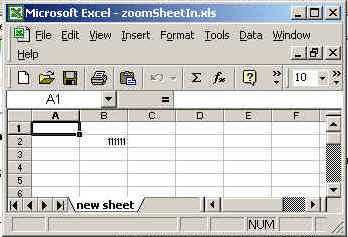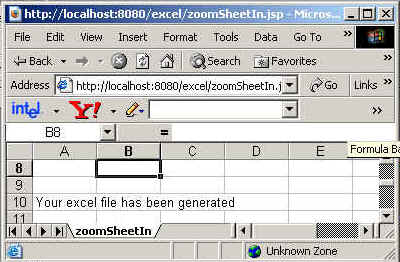Tutorials

# Zoom in Excel

In this program we are going to make a java program through which we can zoon in(-) an excel sheet.

In this program we are going to make a java program through which we can zoon in(-) an excel sheet.

# zoom in excel

In this program we are going to make a java program through which we can zoon in(-)  an excel sheet. By this means you can make an excel sheet smaller than their original size .

The package we need to import is java.io.*,java.util.* ,org.apache.poi.hssf.usermodel.HSSFSheet, org.apache.poi.usermodel.HSSFRow, org.apache.poi.usermodel.HSSFCell and org.apache.poi.hssf.usermodel. HSSFWorkbook.

setZoom(int,int):
This method is used to set the zoom ratio of the excel sheet e.g. setZoom(3,4) means zoom the sheet as 75%.

In this example we are creating the sheet and set the zoom ratio 75%. This means the output of the sheet will be zoomed in 25% of their original size. In other words we can say the size of excel sheet will be shrunken by 25%.

The code of the program is given below:

 ``` <%@ page import="org.apache.poi.hssf.usermodel.HSSFSheet"%> <%@ page import="org.apache.poi.hssf.usermodel.HSSFWorkbook"%> <%@ page import="org.apache.poi.hssf.usermodel.HSSFRow"%> <%@ page import="org.apache.poi.hssf.usermodel.HSSFCell"%> <%@ page contentType="application/vnd.ms-excel" %> <%@ page import="java.io.*" %> <%@ page import="java.util.*" %> <%try{   HSSFWorkbook hwb = new HSSFWorkbook();   HSSFSheet sheet = hwb.createSheet("new sheet");   HSSFRow row = sheet.createRow((short)1);   HSSFCell cell = row.createCell((short)1);   cell.setCellValue(111111);     sheet.setZoom(3,4);   FileOutputStream fileOut = new FileOutputStream ("c:\\excel\\zoomSheetIn.xls");   hwb.write(fileOut);   fileOut.close();   out.println("Your excel file has been generated");  } catch ( Exception ex ) {    } %>```

The output of the program is given below: